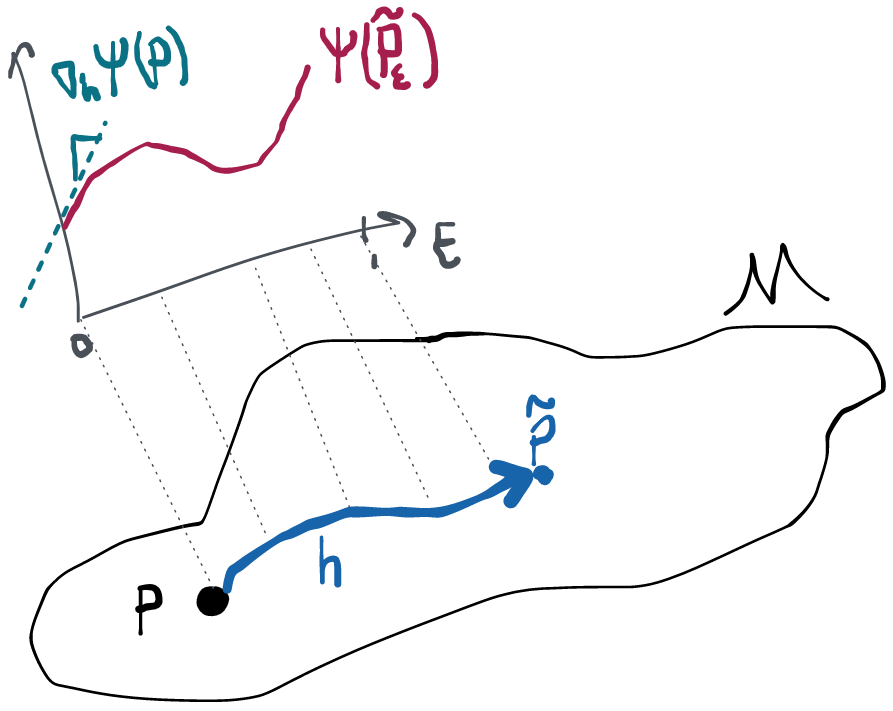Comment
Search
Duplicate
Try NotionDrag image to reposition
3. Efficiency Theory
Chapter 2 was all about making our inference causal. But now we’re done with causality. We have a statistical estimand, which is a property of a distribution we can observe data from, and we want to estimate it. How do we build an estimator that, with enough data, a) converges to the right answer and b) has a known sampling distribution so we can get confidence intervals and p-values?
In this chapter we’ll take a step back from the estimator construction problem and consider an easier problem: if someone were to give you several estimators that all had the above properties, how would you pick which one to use? To answer this we have to define a useful notion of optimality. Then we will see in chapter 4 that there are several nice ways that we can construct such optimal estimators.
In terms of deciding on which estimator we should use, we’ll follow this roadmap:
First we'll lay out our "dealbreaker" conditions- any estimator that doesn't meet these will be automatically discarded.
Among the estimators that remain, we will order them based on a continuous performance metric and choose the one that is best.
Understanding this process is the focus of the next two sections. We’ll see that something called the efficient influence function is central to optimality. In the third section in this chapter we will therefore turn our attention to practical methods you can use to derive efficient influence functions.
Credit Due
Efficiency theory has a rich history of many ideas building on top of each other over time and being re-contextualized to new uses. My own understanding of the theory as it applies to efficient estimation comes primarily from lectures given by Mark van der Laan at UC Berkeley, as well as the wonderful books by Anastasios Tsiatis and Aad van der Vaart. Another canonical (albeit dense) reference is van der Laan and Robins. For influence function derivation techniques I am indebted to Oliver Hines and Edward Kennedy for their practical approaches in nonparametric models and once again to Anastasios Tsiatis and lectures by Mark van der Laan for useful approaches in semiparametric models.
sections in this chapter